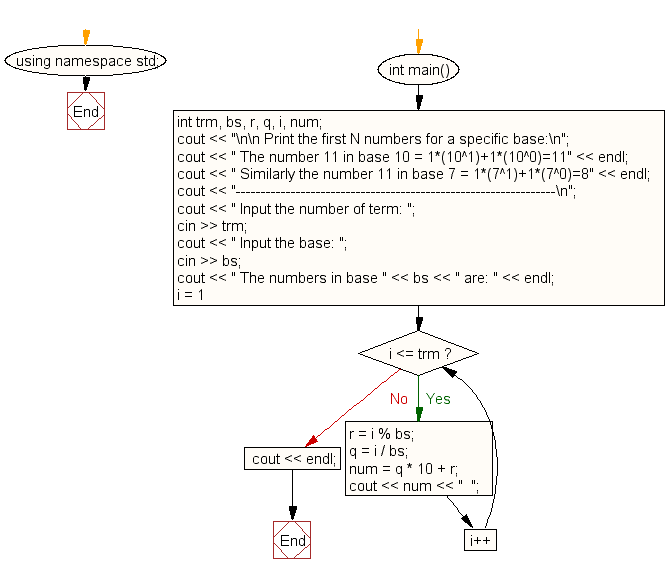﻿ C++ Exercises: Print the first N numbers for a specific base - w3resource# C++ Exercises: Print the first N numbers for a specific base

## C++ For Loop: Exercise-68 with Solution

Write a program that will print the first N numbers for a specific base.

Sample Solution:-

C++ Code :

``````#include <iostream>
using namespace std;
int main()
{
int trm, bs, r, q, i, num;
cout << "\n\n Print the first N numbers for a specific base:\n";
cout << " The number 11 in base 10 = 1*(10^1)+1*(10^0)=11" << endl;
cout << " Similarly the number 11 in base 7 = 1*(7^1)+1*(7^0)=8" << endl;
cout << "----------------------------------------------------------------\n";
cout << " Input the number of term: ";
cin >> trm;
cout << " Input the base: ";
cin >> bs;
cout << " The numbers in base " << bs << " are: " << endl;
for (i = 1; i <= trm; i++)
{
r = i % bs;
q = i / bs;
num = q * 10 + r;
cout << num << "  ";
}
cout << endl;
}
``````

Sample Output:

``` Print the first N numbers for a specific base:
The number 11 in base 10 = 1*(10^1)+1*(10^0)=11
Similarly the number 11 in base 7 = 1*(7^1)+1*(7^0)=8
----------------------------------------------------------------
Input the number of term: 15
Input the base: 9
The numbers in base 9 are:
1  2  3  4  5  6  7  8  10  11  12  13  14  15  16
```

Flowchart:C++ Code Editor:

Contribute your code and comments through Disqus.

What is the difficulty level of this exercise?

﻿

## C++ Programming: Tips of the Day

What is a smart pointer and when should I use one?

This answer is rather old, and so describes what was 'good' at the time, which was smart pointers provided by the Boost library. Since C++11, the standard library has provided sufficient smart pointers types, and so you should favour the use of std::unique_ptr, std::shared_ptr and std::weak_ptr.

There was also std::auto_ptr. It was very much like a scoped pointer, except that it also had the "special" dangerous ability to be copied - which also unexpectedly transfers ownership.

It was deprecated in C++11 and removed in C++17, so you shouldn't use it.

```std::auto_ptr<MyObject> p1 (new MyObject());
std::auto_ptr<MyObject> p2 = p1; // Copy and transfer ownership.
// p1 gets set to empty!
p2->DoSomething(); // Works.
p1->DoSomething(); // Oh oh. Hopefully raises some NULL pointer exception.
```

Ref : https://bit.ly/3mc9GHE